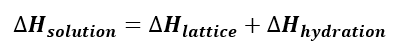# Problem: Use the data below to calculate the heat of hydration of lithium chloride.Compound Lattice energy  (kJ/mol) ΔHsoln (kJ/mol)LiCl-834-37.0NaCl-769+3.88

###### FREE Expert Solution

When we are calculating for the heat of solution, we are using the following equation:Lithium Chloride: LiCl

88% (449 ratings)###### Problem Details

Use the data below to calculate the heat of hydration of lithium chloride.

 Compound Lattice energy  (kJ/mol) ΔHsoln (kJ/mol) LiCl -834 -37.0 NaCl -769 +3.88